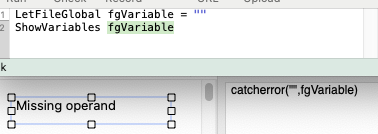# CatchError( and Evaluate Formula Twice

It’s hardly a high priority item since it is highly circumstantial, but I would expect catch error( to work here.

With a Text Display set to Evaluate Formula Twice, if the variable containing the data for the formula is empty it does not suppress the error.I think the problem is that the error occurs after the execution of the catcherror( function. If the second parameter of a catcherror( function is an error, then catcherror( returns its first parameter. It’s not designed to catch any other errors.

If fgVariable doesn’t exist, or hasn’t been assigned an initial value, then catcherror( will catch that error, and return the first operand, which is a blank. The Missing operand error is because the second evaluation is trying to evaluate that blank.

You could use `catcherror({""},fgVariable)`, and that should work if the variable is undefined, or hasn’t been initialized. It won’t work if the variable was initialized to something that isn’t a valid formula, because then there is no error in reading that variable. The error would be in trying to evaluate that invalid formula.

You’re exactly right.

In my use, the variable is deliberately defined as empty at times, so I can’t suppress the error message.

Instead of defining it as empty, define it like this.

``````fgVariable = {""}
``````

That way, you have it set to a valid formula that returns a blank. There is no error to suppress.

Brilliant, David. Thanks

I think David’s solution is probably the best.

Here is some additional context on the Evaluate Formula Twice option. Early versions of Panorama limited the Text Display formula to 255 characters. I can’t remember if this limit continued all the way thru Panorama 6. In Panorama X, there is no limit to the formula length.

The Evaluate Formula Twice option was conceived as a hack to get around this 255 character limit. You could put a formula of any length into a variable, then use that variable with the Evaluate Formula Twice option so that a very long formula could be used. It was inconvenient but if you needed an extra long formula at least it wasn’t impossible.

Panorama X doesn’t have the 255 character limit, so this hack isn’t needed for this purpose any more. And really, the Evaluate Formula Twice option isn’t needed at all any more, because you could use the formulavalue( function to get the same effect. So instead of enabling the Evaluate Formula Twice option, Jim cook use this formula:

``````catcherror("",formulavalue(fgVariable))
``````

In that case, you could set fgVariable to `{""}` or `""`, it wouldn’t really matter. The latter case causes a missing operand error, but the catcherror( function catches it.

I knew there was something like formulavalue( but couldn’t find it. Knowing Evaluate Formula Twice from past use it seemed like an easy alternative. Now I know better.